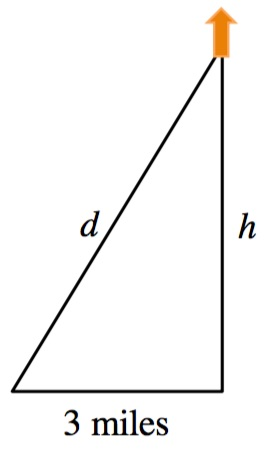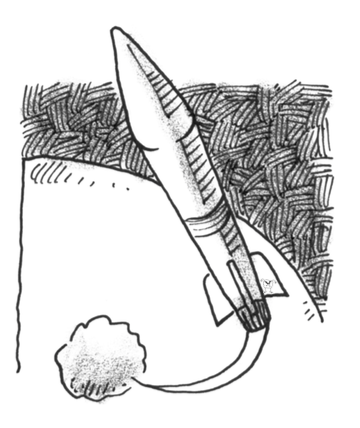### Home > APCALC > Chapter 10 > Lesson 10.2.1 > Problem10-115

10-115.

A rocket, rising vertically, is being tracked by a radar station $3$ miles away from the rocket’s launch pad. How fast is the rocket rising when it is $5$ miles above the ground and the distance between the rocket and the radar station is increasing at a rate of $3000$ miles per hour?

Draw a diagram and use it to write an equation.Differentiate your equation from Step 1.

What is the value of d when $h = 5$?

Evaluate your equation from Step 2 for $h = 5$, $d = \text{(# from Step 3)}$, and $d^\prime = 3000$.